Mean or Expectation of random variable

Chapter 13 Class 12 Probability (Term 2)
Concept wise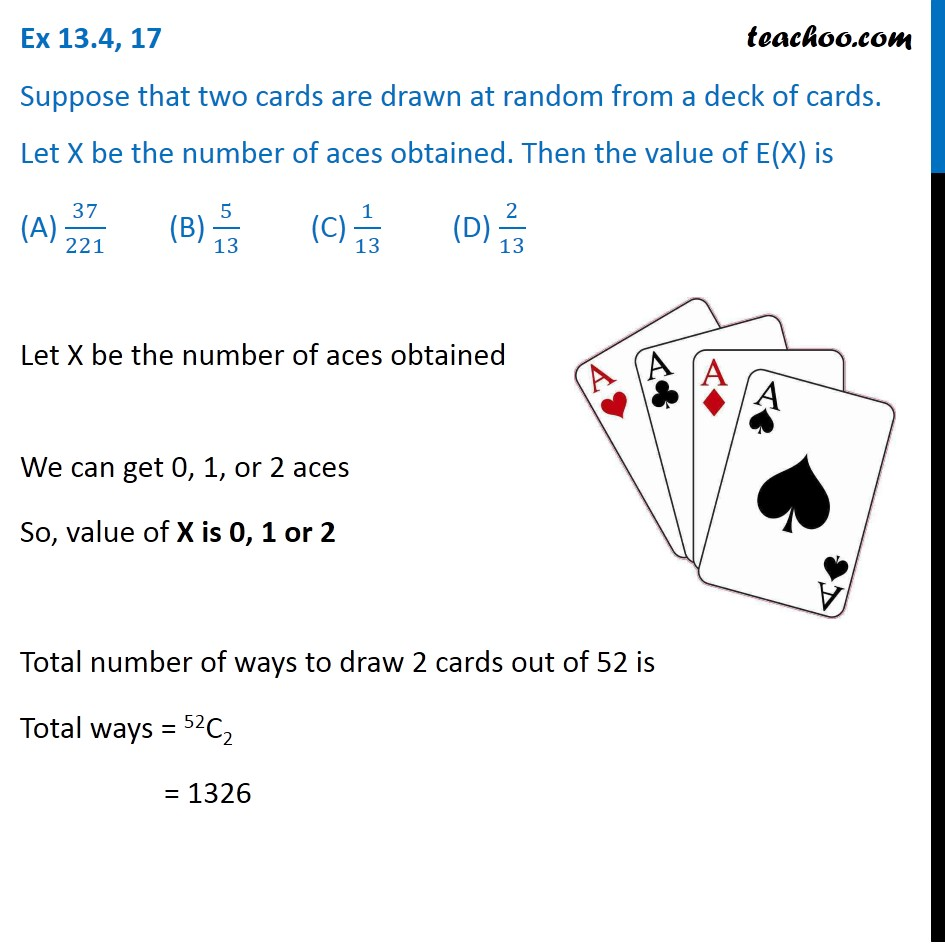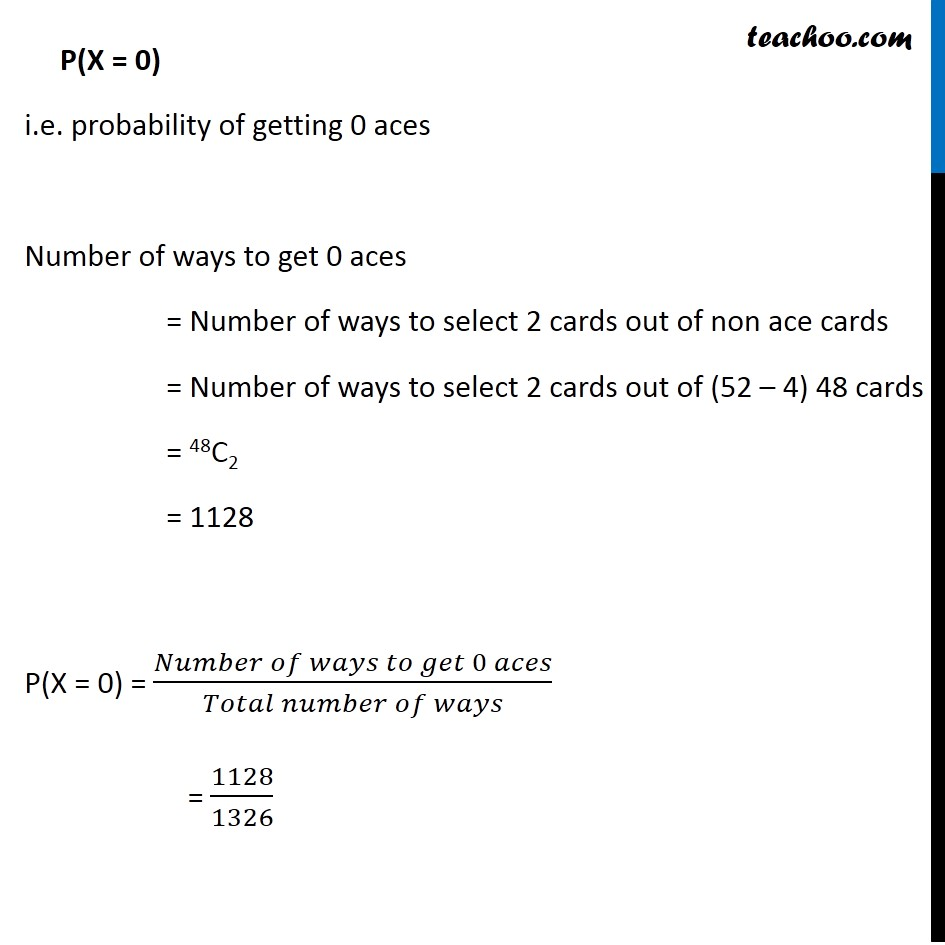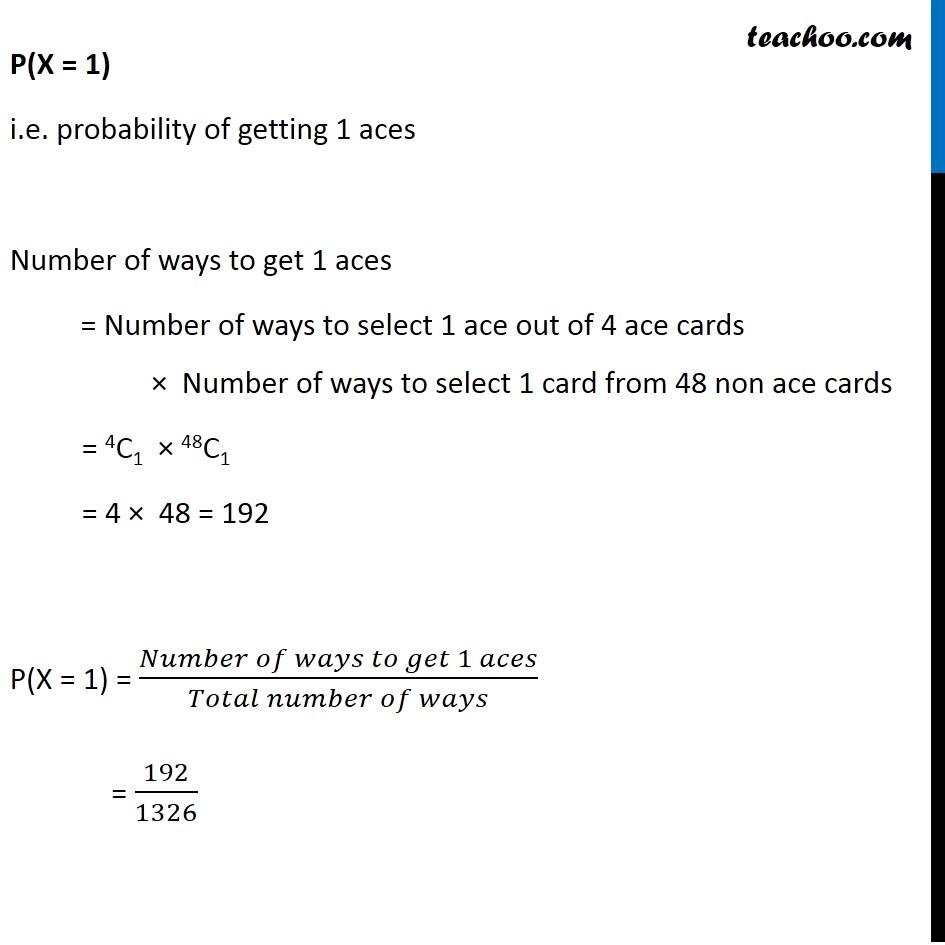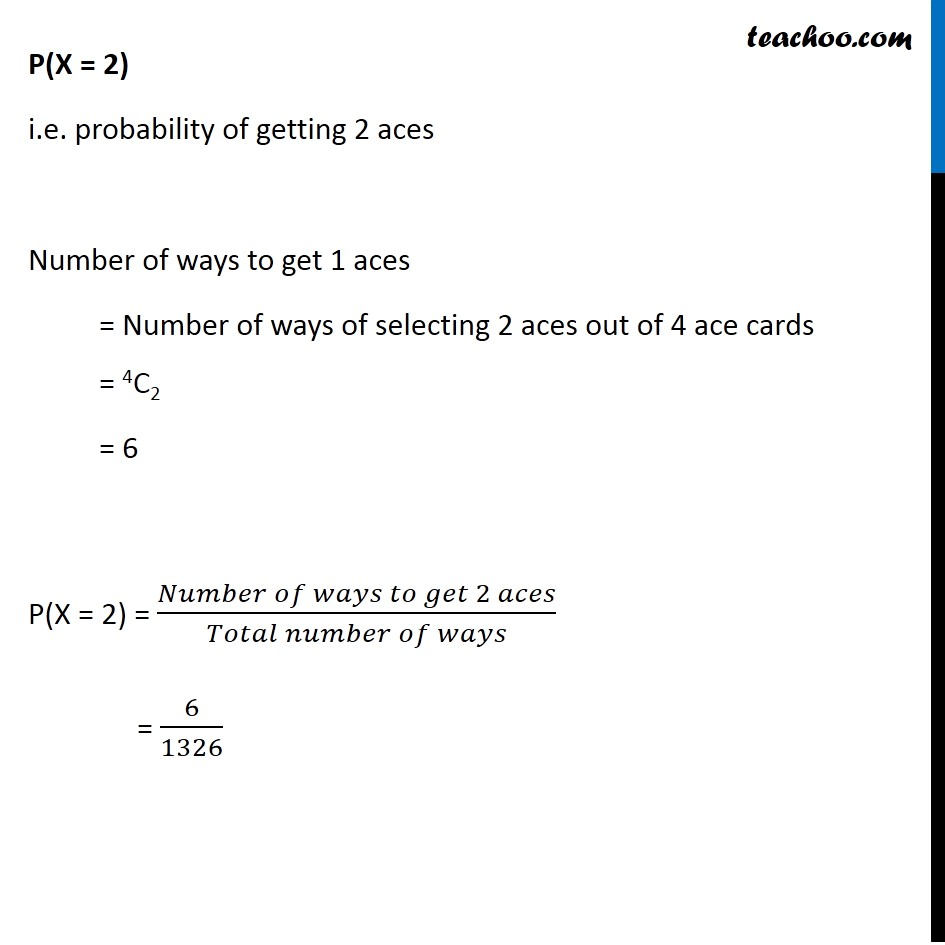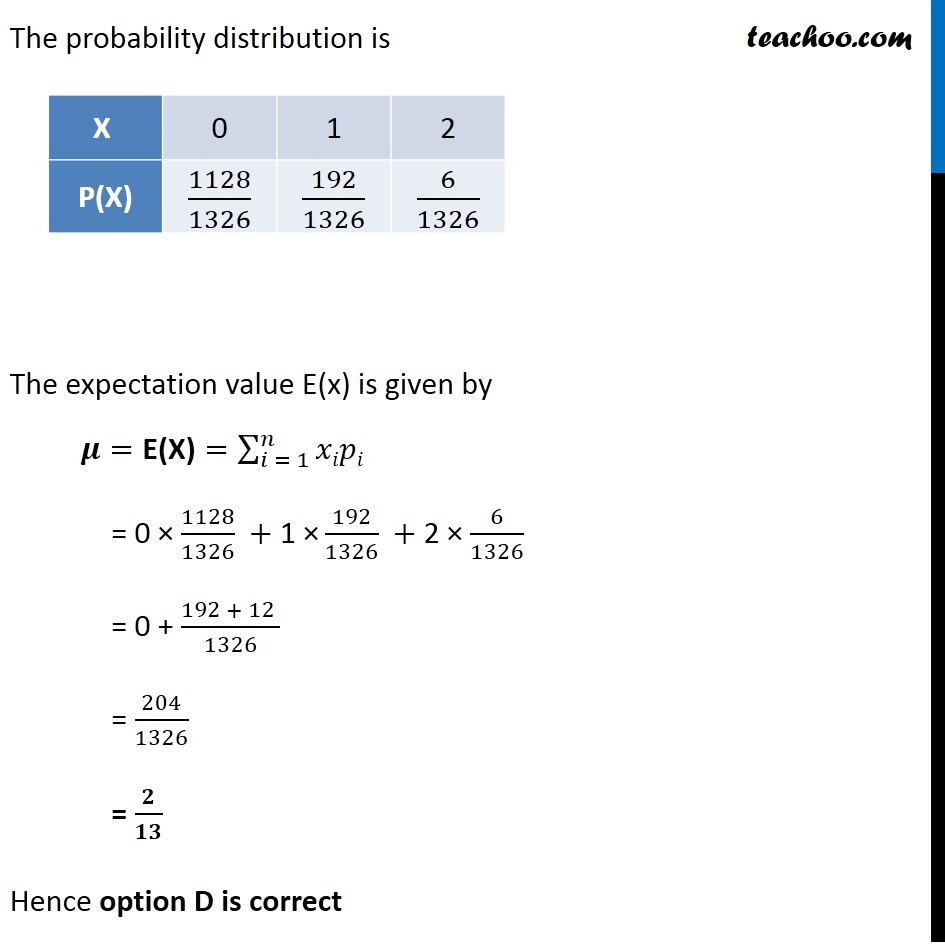### Transcript

Ex 13.4, 17 Suppose that two cards are drawn at random from a deck of cards. Let X be the number of aces obtained. Then the value of E(X) is (A) 37/221 (B) 5/13 (C) 1/13 (D) 2/13Let X be the number of aces obtained We can get 0, 1, or 2 aces So, value of X is 0, 1 or 2 Total number of ways to draw 2 cards out of 52 is Total ways = 52C2 = 1326 P(X = 0) i.e. probability of getting 0 aces Number of ways to get 0 aces = Number of ways to select 2 cards out of non ace cards = Number of ways to select 2 cards out of (52 – 4) 48 cards = 48C2 = 1128 P(X = 0) = (𝑁𝑢𝑚𝑏𝑒𝑟 𝑜𝑓 𝑤𝑎𝑦𝑠 𝑡𝑜 𝑔𝑒𝑡 0 𝑎𝑐𝑒𝑠)/(𝑇𝑜𝑡𝑎𝑙 𝑛𝑢𝑚𝑏𝑒𝑟 𝑜𝑓 𝑤𝑎𝑦𝑠) = 1128/1326 P(X = 1) i.e. probability of getting 1 aces Number of ways to get 1 aces = Number of ways to select 1 ace out of 4 ace cards × Number of ways to select 1 card from 48 non ace cards = 4C1 × 48C1 = 4 × 48 = 192 P(X = 1) = (𝑁𝑢𝑚𝑏𝑒𝑟 𝑜𝑓 𝑤𝑎𝑦𝑠 𝑡𝑜 𝑔𝑒𝑡 1 𝑎𝑐𝑒𝑠)/(𝑇𝑜𝑡𝑎𝑙 𝑛𝑢𝑚𝑏𝑒𝑟 𝑜𝑓 𝑤𝑎𝑦𝑠) = 192/1326 P(X = 2) i.e. probability of getting 2 aces Number of ways to get 1 aces = Number of ways of selecting 2 aces out of 4 ace cards = 4C2 = 6 P(X = 2) = (𝑁𝑢𝑚𝑏𝑒𝑟 𝑜𝑓 𝑤𝑎𝑦𝑠 𝑡𝑜 𝑔𝑒𝑡 2 𝑎𝑐𝑒𝑠)/(𝑇𝑜𝑡𝑎𝑙 𝑛𝑢𝑚𝑏𝑒𝑟 𝑜𝑓 𝑤𝑎𝑦𝑠) = 6/1326 The probability distribution is The expectation value E(x) is given by 𝝁="E(X)"=∑2_(𝑖 = 1)^𝑛▒𝑥𝑖𝑝𝑖 = 0 × 1128/1326 +"1 ×" 192/1326 + 2 × 6/1326 = 0 + (192 + 12 )/1326 = 204/1326 = 𝟐/𝟏𝟑 Hence option D is correct